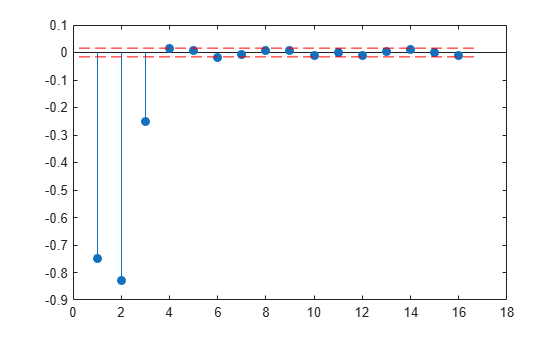# levinson

Levinson-Durbin recursion

## Syntax

``a = levinson(r,n)``
``[a,e,k] = levinson(___)``

## Description

example

````a = levinson(r,n)` returns the coefficients of an autoregressive linear process of order `n` that has `r` as its autocorrelation sequence.```

example

````[a,e,k] = levinson(___)` also returns the prediction error `e`, and the reflection coefficients, `k`.```

## Examples

collapse all

Estimate the coefficients of an autoregressive process given by

`$x\left(n\right)=0.1\phantom{\rule{0.16666666666666666em}{0ex}}x\left(n-1\right)-0.8\phantom{\rule{0.16666666666666666em}{0ex}}x\left(n-2\right)-0.27\phantom{\rule{0.16666666666666666em}{0ex}}x\left(n-3\right)+w\left(n\right).$`

`a = [1 0.1 -0.8 -0.27];`

Generate a realization of the process by filtering white noise of variance 0.4.

```v = 0.4; w = sqrt(v)*randn(15000,1); x = filter(1,a,w);```

Estimate the correlation function. Discard the correlation values at negative lags. Use the Levinson-Durbin recursion to estimate the model coefficients. Verify that the prediction error corresponds to the variance of the input.

```[r,lg] = xcorr(x,'biased'); r(lg<0) = []; [ar,e] = levinson(r,numel(a)-1)```
```ar = 1×4 1.0000 0.0772 -0.7954 -0.2493 ```
```e = 0.3909 ```

Estimate the reflection coefficients for a 16th-order model. Verify that the only reflection coefficients that lie outside the 95% confidence bounds are the ones that correspond to the correct model order. See AR Order Selection with Partial Autocorrelation Sequence for more details.

```[~,~,k] = levinson(r,16); stem(k,'filled') conf = sqrt(2)*erfinv(0.95)/sqrt(15000); hold on [X,Y] = ndgrid(xlim,conf*[-1 1]); plot(X,Y,'--r') hold off```Generate the coefficients of an autoregressive process given by

`$x\left(n\right)=0.1\phantom{\rule{0.16666666666666666em}{0ex}}x\left(n-1\right)-0.8\phantom{\rule{0.16666666666666666em}{0ex}}x\left(n-2\right)-0.27\phantom{\rule{0.16666666666666666em}{0ex}}x\left(n-3\right)+w\left(n\right).$`

`a = [1 0.1 -0.8 -0.27];`

Generate five realizations of the process by filtering white noise with different variances.

```nr = 5; v = rand(1,nr)```
```v = 1×5 0.8147 0.9058 0.1270 0.9134 0.6324 ```
```w = sqrt(v).*randn(15000,nr); x = filter(1,a,w);```

Estimate the correlation function. Discard cross-correlation terms and correlation values at negative lags. Use the Levinson-Durbin recursion to estimate the prediction errors for the correct model order and verify that the prediction errors correspond to the variances of the input noise signals.

```[r,lg] = xcorr(x,'biased'); [~,e] = levinson(r(lg>=0,1:nr+1:end),numel(a)-1)```
```e = 5×1 0.7957 0.9045 0.1255 0.9290 0.6291 ```

## Input Arguments

collapse all

Autocorrelation sequence, specified as a vector or matrix. If `r` is a matrix, the function finds the coefficients for each column of `r` and returns them in the rows of `a`.

Example: `[r,lg] = xcorr(randn(1000,1),'biased'); r(lg<0) = []` estimates the autocorrelation sequence of a 1000-sample random signal for positive lags.

Data Types: `single` | `double`
Complex Number Support: Yes

Model order, specified as a positive integer scalar.

Data Types: `single` | `double`

## Output Arguments

collapse all

Autoregressive linear process coefficients, returned as a row vector or matrix. The filter coefficients are ordered in descending powers of z–1:

`$H\left(z\right)=\frac{1}{A\left(z\right)}=\frac{1}{1+a\left(2\right){z}^{-1}+\cdots +a\left(n+1\right){z}^{-n}}.$`

If `r` is a matrix, then each row of `a` corresponds to a column of `r`.

Prediction error, returned as a scalar or column vector. If `r` is a matrix, then each element of `e` corresponds to a column of `r`.

Reflection coefficients, returned as a column vector of length `n`. If `r` is a matrix, then each column of `k` corresponds to a column of `r`.

Note

`k` is computed internally while computing the `a` coefficients, so returning `k` simultaneously is more efficient than converting `a` to `k` with `tf2latc`.

## Algorithms

The Levinson-Durbin recursion is an algorithm for finding an all-pole IIR filter with a prescribed deterministic autocorrelation sequence. It has applications in filter design, coding, and spectral estimation. The filter that `levinson` produces is minimum phase.

`levinson` solves the symmetric Toeplitz system of linear equations

where `r = [`r(1) ... r(n + 1)`]` is the input autocorrelation vector, and r(i)* denotes the complex conjugate of r(i). The input `r` is typically a vector of autocorrelation coefficients where lag 0 is the first element, r(1).

Note

If `r` is not a valid autocorrelation sequence, the `levinson` function might return `NaN`s even if the solution exists.

The algorithm requires O(n2) flops and is thus much more efficient than the MATLAB® backslash command for large `n`. However, the `levinson` function uses `\` for low orders to provide the fastest possible execution.

 Ljung, Lennart. System Identification: Theory for the User. 2nd Ed. Upper Saddle River, NJ: Prentice Hall, 1999.